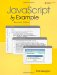# EXERCISES

 1: In the expression 6 + 4 / 2 % 2 what are the operands and in what order are they evaluated? Show operator preference by using parentheses. 2: How can JavaScript tell if the + is used for concatenation or addition? Write a short program to demonstrate . 3: If x is assigned the value of 5 , what is y in the following two statements: ` ` ` y = --x; y = x--; ` Explain the output of the preceding two statements. 4: Are the following true or false: ` ` ` 22 == "22" 22 === "22" "2" > "100" ` Write a script to prove your answers to the preceding. In the same script, use the following two statements: ` ` ` document.write("3" + "4"); document.write(3 + 4); ` Explain the output of the two preceding statements. 5: Example 5.8 prompts the user for his age. The user 's response is assigned to the variable answer as a string value. Rewrite the program to assure that the age entered is a number before testing it. How do you do this? 6: Ask the user for a Fahrenheit temperature, and then convert it to Celsius. Use parseFloat() . To specify the precision of the number, see "The Number Object" on page 190 in Chapter 9. Formula for conversion: C = 5/9(F “ 32). 7: The user is visiting Thailand. He has 65 U.S. dollars. Tell him how many baht that amounts to. There are approximately 42 baht to a U.S. dollar. Display an image of the Thai flag on the same page.JavaScript by Example (2nd Edition)
ISBN: 0137054890
EAN: 2147483647
Year: 2003
Pages: 150
Authors: Ellie Quigley

Similar book on Amazon